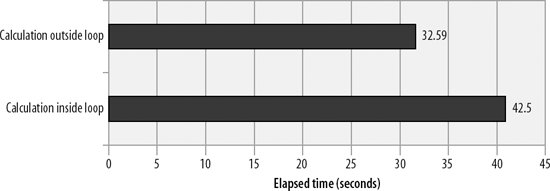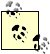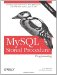# Optimizing Loops

In the remainder of this chapter we will look at techniques for the optimization of stored program code that does not involve SQL statements, starting with the optimization of loops .

Because the statements executed within a loop can be executed many times, optimizing loop processing is a basic step when optimizing the performance of a program written in any language. The MySQL stored program language is no exception.

22.5.1. Move Unnecessary Statements Out of a Loop

The first principle of optimizing a loop is to move calculations out of the loop that don't belong inside the loop (these are known as loop-invariant statements , since they do not vary with each execution of the loop body). Although such a step might seem obvious, it's surprising how often a program will perform calculations over and over within a loop that could have been performed just once before the start of loop execution.

For instance, consider the stored program in Example 22-9. This loop is actually fairly inefficient, but at first glance it's not easy to spot where the problem is. Fundamentally, the problem with this stored program is that it calculates the square root of the i variable for every value of the j variable. Although there are only 1,000 different values of i, the stored program calculates this square root five million times.

Example 22-9. A poorly constructed loop

 ``` WHILE (i<=1000) DO SET j=1; WHILE (j<=5000) DO SET rooti=sqrt(i); SET rootj=sqrt(j); SET sumroot=sumroot+rooti+rootj; SET j=j+1; END WHILE; SET i=i+1; END WHILE; ```

By moving the calculation of the square root of i outside of the loopas shown in Example 22-10we substantially reduce the overhead of this loop.

Example 22-10. Moving unnecessary calculations out of a loop

 ``` WHILE (i<=1000) DO SET rooti=sqrt(i); SET j=1; WHILE (j<=5000) DO SET rootj=sqrt(j); SET sumroot=sumroot+rootj+rooti; SET j=j+1; END WHILE; SET i=i+1; END WHILE; ```

Figure 22-5 shows the performance improvements achieved from moving the calculation of the square root of the i variable outside of the inner loop.

Figure 22-5. Performance improvements gained from removing unnecessary calculations within a loopEnsure that all statements within a loop truly belong within the loop. Move any loop-invariant statements outside of the loop.

22.5.2. Use LEAVE or CONTINUE to Avoid Needless Processing

Just as it is important to remove all unnecessary processing from a loop, it is equally important to leave the loop when you are finished. Again, this seems obvious, but it is easy to write a fully functional loop that performs unnecessary iterations. When you look at your code, it's not always that obvious that the loop is inefficient.

Consider the loop shown in Example 22-11: this is a variation on the loop used in Example 22-1 to count prime numbers. This loop is functionally correct, but inefficient. On line 2 we cycle through all numbers less than the given number looking for divisors. If we find a divisor (line 4), we know that the number is not a prime number. However, in Example 22-11, we continue to check each number even though we have already found the first divisor. This is unnecessary, since once we find even a single divisor, we know that the number is not primethere is no need to look for further divisors.

Example 22-11. Loop that iterates unnecessarily

 ```1 divisor_loop: 2 WHILE (j ```

Example 22-12 shows the same loop, but with a LEAVE statement added that terminates the loop once a divisor is found.

Example 22-12. Loop with a LEAVE statement to avoid unnecessary iterations

 ``` divisor_loop: WHILE (jLEAVE divisor_loop; /* No need to keep checking*/ END IF; SET j=j+1; END WHILE ; ```

Although the LEAVE statement terminates the loop and reduces the elapsed time for the stored procedure, it may decrease readability of the code because the loop now has two sections that determine if the loop continuesthe WHILE clause condition and the LEAVE statement. Constructing a loop with multiple exit points makes the code harder to understand and maintain.

It would be equally valid in this case to modify the WHILE clause so that the loop ceases its repetitions once it has determined that the number is not a prime, as shown in Example 22-13.

Example 22-13. Modifying the WHILE condition to avoid unnecessary iterations

 ``` divisor_loop: WHILE (jAND isprime=1) do /* Look for a divisor */ IF (MOD(i,j)=0) then SET isprime=0; /* This number is not prime*/ END IF; SET j=j+1; END WHILE ; ```

Figure 22-6 shows the improvements gained in our prime number search when we add the LEAVE statement or modify the WHILE clause. Modifying the WHILE clause leads to a comparable performance increase without reducing the readability of the loop.

Figure 22-6. Effect of using LEAVE or modifying WHILE clause to avoid unnecessary iterationsMake sure that your loops terminate when all of the work has been done, either by ensuring that the loop continuation expression is comprehensive orif necessaryby using a LEAVE statement to terminate when appropriate.MySQL Stored Procedure Programming
ISBN: 0596100892
EAN: 2147483647
Year: 2004
Pages: 208